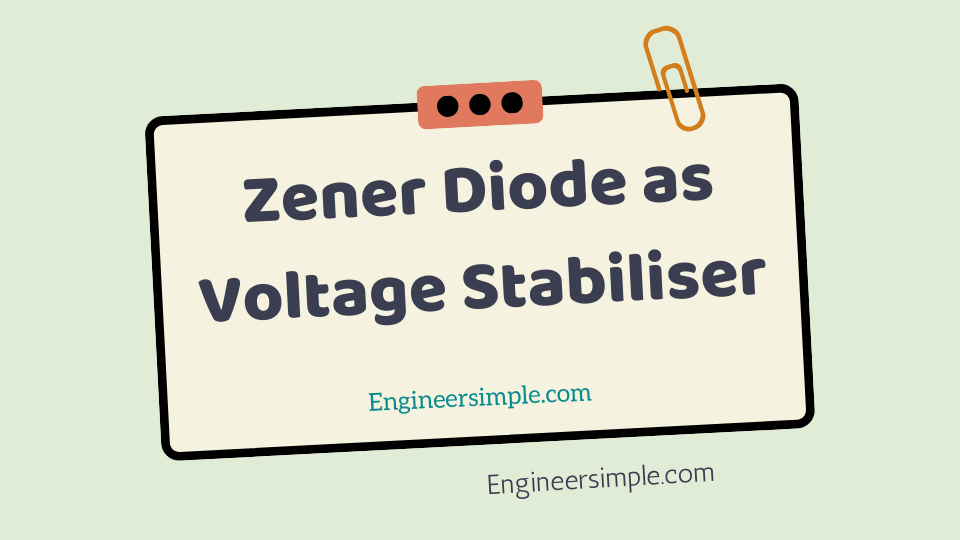Zener Diode as Voltage Stabiliser - Engineer Simple

#A zener diode can be used as a voltage regulator to provide a constant voltage from a
source whose voltage may vary over sufficient range. The circuit arrangement is shown
in Fig.1.

The zener diode of zener voltage Vz is reverse connected across which
constant output is desired. The series resistance R absorbs the output voltage
fluctuations so as to maintain constant voltage across the load. It may be noted that the
zener will maintain a constant voltage V z (=E 0 ) across the load so long as the input
voltage does not fall below Vz.
When the circuit is properly designed, the load voltage E 0 remains essentially
constant (equal to V z ) even though the input voltage E 1 and load resistance R L may vary
over a wide range.

##### (ii). It is clear that output voltage remains constant at V z (=E 0 ). The excessvoltage is f=drop across the series resistance R. This will cause anload current remains constant. Hence, output voltage E 0 remains constantirrespective of the changes in the input voltage E 1.

(ii) Now suppose that input voltages constant but the load resistance R L
decreases. This will cause an increase in load current. The extra current
cannot come from the source because drop in R (and hence source
current I) will not change as the zener is within its regulating range. The
additional load current will come from a decrease in zener current Iz.
Consequently, the output voltage stays at constant value.
Voltage frop across R = E1 – E0
Current through R, I = Iz + I L
Applying Ohm’s law, we have,
R =( E1 – E0 )/( Iz + I L )